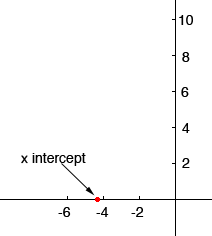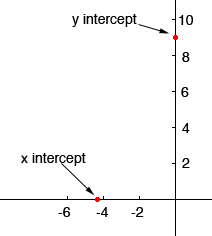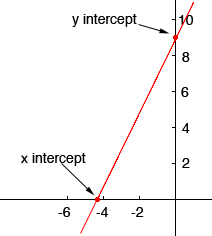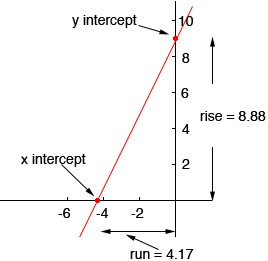Hi my name is anthony, the level is middle, and i am a student. I have a question to help me study for a math exam coming up on january 30. Here is the question, and i don't know how to graph it either, so if you can please include a picture or web site that shows the labeled graph: Given -1.7x + 0.8y=7.1: (a) state the intercepts and slope for the graph (b) Draw the graph Hi Anthony, To find the x intercept you need to find where the graph crosses the x-axis. All the points on the x-axis have their y-coordinate equal to zero. Thus, in the equation, set y = 0 and solve for x. -1.7x + 0.8y = 7.1 set y = 0 -1.7x + 0.80 = 7.1 -1.7x = 7.1 y = 7.1/-1.7 = - 4.17 Thus the x intercept is - 4.17To find the y intercept you need to find where the graph crosses the y-axis. All the points on the y-axis have their x-coordinate equal to zero. Thus, in the equation, set x = 0 and solve for y. -1.7x + 0.8y = 7.1 set x = 0 -1.70 + 0.8y = 7.1 0.8y = 7.1 y = 7.1/0.8 = 8.88 Thus the y intercept is 8.88Now that you know two points on the graph you can use a ruler and draw the graphFinally, the slope you can find two ways. The first method is to read the rise and run from the graph.So the slope is m = 8.88/4.17 = 2.1 The second method is to use the equation. To do this you need to put the equation into the form y = m x + b and then m is the slope. The equation you have is -1.7x + 0.8y = 7.1 add 1.7x to both sides to get 0.8 y = 1.7 x + 7.1 Now divide both sides by 0.8 to get y = 2.1 x + 8.9 Thus the slope is 2.1 I hope this helps, Penny